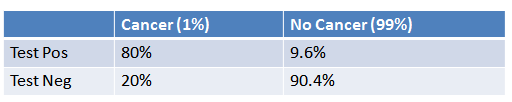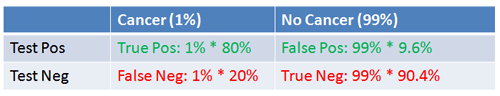# Why can a test for breast cancer with a supposed very high positive accuracy rate yield in reality, a tenth of the accuracy?

Please bear in mind that this is simply to examine probability outcomes and the biases we have when faced with a problem when deducing probabilistic outcomes. There are many medical variables to take into account with medical tests of any kind and this article doesn’t include outcome variance in age, environmental contributing factors, hereditary factors etc.

Bayes’ theorem was the subject of a detailed article. The essay is good, but over 15,000 words long — here’s the condensed version for Bayesian newcomers like myself:

• Tests are not the event. We have a cancer test, separate from the event of actually having cancer. We have a test for spam, separate from the event of actually having a spam message.
• Tests are flawed. Tests detect things that don’t exist (false positive), and miss things that do exist (false negative).
• Tests give us test probabilities, not the real probabilities. People often consider the test results directly, without considering the errors in the tests.
• False positives skew results. Suppose you are searching for something really rare (1 in a million). Even with a good test, it’s likely that a positive result is really a false positive on somebody in the 999,999.
• People prefer natural numbers. Saying “100 in 10,000″ rather than “1%” helps people work through the numbers with fewer errors, especially with multiple percentages (“Of those 100, 80 will test positive” rather than “80% of the 1% will test positive”).
• Even science is a test. At a philosophical level, scientific experiments can be considered “potentially flawed tests” and need to be treated accordingly. There is a test for a chemical, or a phenomenon, and there is the event of the phenomenon itself. Our tests and measuring equipment have some inherent rate of error.

Bayes’ theorem converts the results from your test into the real probability of the event. For example, you can:

• Correct for measurement errors. If you know the real probabilities and the chance of a false positive and false negative, you can correct for measurement errors.
• Relate the actual probability to the measured test probability. Bayes’ theorem lets you relate Pr(A|X), the chance that an event A happened given the indicator X, and Pr(X|A), the chance the indicator X happened given that event A occurred. Given mammogram test results and known error rates, you can predict the actual chance of having cancer.

## Anatomy of a Test

The article describes a cancer testing scenario:

• 1% of women have breast cancer (and therefore 99% do not).
• 80% of mammograms detect breast cancer when it is there (and therefore 20% miss it).
• 9.6% of mammograms detect breast cancer when it’s not there (and therefore 90.4% correctly return a negative result).

Put in a table, the probabilities look like this:• 1% of people have cancer
• If you already have cancer, you are in the first column. There’s an 80% chance you will test positive. There’s a 20% chance you will test negative.
• If you don’t have cancer, you are in the second column. There’s a 9.6% chance you will test positive, and a 90.4% chance you will test negative.

## How Accurate Is The Test?

Now suppose you get a positive test result. What are the chances you have cancer? 80%? 99%? 1%?

Here’s how I think about it:

• Ok, we got a positive result. It means we’re somewhere in the top row of our table. Let’s not assume anything — it could be a true positive or a false positive.
• The chances of a true positive = chance you have cancer * chance test caught it = 1% * 80% = .008
• The chances of a false positive = chance you don’t have cancer * chance test caught it anyway = 99% * 9.6% = 0.09504

The table looks like this:And what was the question again? Oh yes: what’s the chance we really have cancer if we get a positive result. The chance of an event is the number of ways it could happen given all possible outcomes:

Probability = desired event / all possibilities

The chance of getting a real, positive result is .008. The chance of getting any type of positive result is the chance of a true positive plus the chance of a false positive (.008 + 0.09504 = .10304).

So, our chance of cancer is .008/.10304 = 0.0776, or about 7.8%.

Interesting — a positive mammogram only means you have a 7.8% chance of cancer, rather than 80% (the supposed accuracy of the test). It might seem strange at first but it makes sense: the test gives a false positive 9.6% of the time, so there will be a ton of false positives in any given population. There will be so many false positives, in fact, that most of the positive test results will be wrong.

Let’s test our intuition by drawing a conclusion from simply eyeballing the table. If you take 100 people, only 1 person will have cancer (1%), and they’re nearly guaranteed to test positive (80% chance). Of the 99 remaining people, about 10% will test positive, so we’ll get roughly 10 false positives. Considering all the positive tests, just 1 in 11 is correct, so there’s a 1/11 chance of having cancer given a positive test. The real number is 7.8% (closer to 1/13, computed above), but we found a reasonable estimate without a calculator.

## Bayes’ Theorem

We can turn the process above into an equation, which is Bayes’ Theorem. It lets you take the test results and correct for the “skew” introduced by false positives. You get the real chance of having the event. Here’s the equation:$\displaystyle{\Pr(\mathrm{A}|\mathrm{X}) = \frac{\Pr(\mathrm{X}|\mathrm{A})\Pr(\mathrm{A})}{\Pr(\mathrm{X|A})\Pr(\mathrm{A})+ \Pr(\mathrm{X | not \ A})\Pr(\mathrm{not \ A})}}$

And here’s the decoder key to read it:

• Pr(A|X) = Chance of having cancer (A) given a positive test (X). This is what we want to know: How likely is it to have cancer with a positive result? In our case it was 7.8%.
• Pr(X|A) = Chance of a positive test (X) given that you had cancer (A). This is the chance of a true positive, 80% in our case.
• Pr(A) = Chance of having cancer (1%).
• Pr(not A) = Chance of not having cancer (99%).
• Pr(X|not A) = Chance of a positive test (X) given that you didn’t have cancer (~A). This is a false positive, 9.6% in our case.

It all comes down to the chance of a true positive result divided by the chance of any positive result. We can simplify the equation to:$\displaystyle{\Pr(\mathrm{A}|\mathrm{X}) = \frac{\Pr(\mathrm{X}|\mathrm{A})\Pr(\mathrm{A})}{\Pr(\mathrm{X})}}$

Pr(X) is a normalizing constant and helps scale our equation. Without it, we might think that a positive test result gives us an 80% chance of having cancer.

Pr(X) tells us the chance of getting any positive result, whether it’s a real positive in the cancer population (1%) or a false positive in the non-cancer population (99%). It’s a bit like a weighted average, and helps us compare against the overall chance of a positive result.

In our case, Pr(X) gets really large because of the potential for false positives. Thank you, normalizing constant, for setting us straight! This is the part many of us may neglect, which makes the result of 7.8% counter-intuitive.